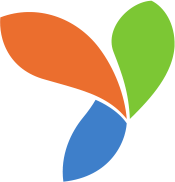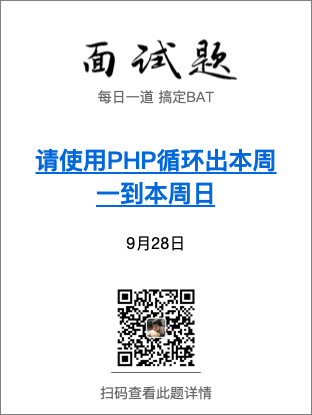# 在yii2应用中，使用imagine库生成分享图实战。# 准备阶段

• imagine图片库
• 二维码生成库
• 一个好看的字体
• 一张海报（自行用PS处理）

## imagine

imagine 支持三种底层的图像处理库（GD、Imagick和Gmagick），GD是最老的图片库，自然处理能力也不如另外两种，本次我使用Imagick作为底层支持，关于PHP如何安装Imagick扩展可参考 https://www.cnblogs.com/aini521521/p/8398770.html

``````composer require imagine/imagine
``````

## 二维码

qrcode-library的安装也非常简单，依然是composer。

``````composer require 2amigos/qrcode-library
``````

# 写入标题

``````use Imagine\Imagick\Imagine;

public function actionShare(\$id){
\$imagine = new Imagine();
//VarDumper::dump(\$image,10,true);
}
``````

imagine和image对象都正常输出了。

## 开始第一次写入

• 文本内容是什么
• 用什么字体
• 字号大小
• 字体颜色
• 从哪个坐标开始

``````Yii::getAlias('@app')."/fonts/yahei.ttf";
``````

``````use Imagine\Imagick\Imagine;
use Imagine\Image\Palette\RGB;
use Imagine\Image\Point;

public function actionShare(\$id){
\$imagine = new Imagine();

\$palette = new RGB();
\$color = \$palette->color("000000");

\$point = new Point(0,0);

\$font = \$imagine->font(Yii::getAlias('@app')."/fonts/yahei.ttf",12,\$color);

\$image->draw()->text("请使用PHP循环出本周一到本周日",\$font,\$point);
\$image->show('jpg');
}
``````

``````text(string \$string, AbstractFont \$font, PointInterface \$position, int \$angle = 0, int \$width = null)
``````

``````use Imagine\Image\Palette\RGB;
use Imagine\Image\Point;
``````

``````\$palette = new RGB();
\$color = \$palette->color("000000");
``````

``````\$font = \$imagine->font(Yii::getAlias('@app')."/fonts/yahei.ttf",12,\$color);
``````

``````\$point = new Point(0,0);
``````

• 字体大小
• 坐标位置

``````\$point = new Point(44,230);
\$font = \$imagine->font(Yii::getAlias('@app')."/fonts/yahei.ttf",24,\$color);
``````

## 内容超过边界问题的处理

``````\$font = \$imagine->font(Yii::getAlias('@app')."/fonts/yahei.ttf",24,\$color);
\$text = \$font->wrapText("请使用PHP循环出本周一到本周日",400);
``````

wrapText方法的第二个参数代表文本最大长度，超过了即为换行，然后将wrapText作用后的\$text再传给 image->draw()->text(); 即可。

``````// vendor/imagine/imagine/src/Image/FontInterface.php:59
public function wrapText(\$string, \$maxWidth, \$angle = 0){
\$words = explode(' ', \$string);
foreach (\$words as \$word) {
if (\$currentLine === null) {
\$currentLine = \$word;
} else {
\$testLine = \$currentLine . ' ' . \$word;
\$testbox = \$this->box(\$testLine, \$angle);
if (\$testbox->getWidth() <= \$maxWidth) {
\$currentLine = \$testLine;
} else {
\$lines[] = \$currentLine;
\$currentLine = \$word;
}
}
}
.....
return implode("\n", \$lines);
}
``````

**那对中文怎么办？**没关系，从官方wrapText的方法我们可以改造出子的方法，只不过每个词的话不不再是空格，我进行了如下改造。

``````\$lines = array();
\$maxWidth = 420;
\$currentLine = null;
\$text = "请使用PHP循环出本周一到本周日";
for(\$i = 0;\$i < mb_strlen(\$text,'UTF-8');\$i++){
\$word = mb_substr(\$text,\$i,1,'UTF-8');
if (\$currentLine === null) {
\$currentLine = \$word;
} else {
\$testLine = \$currentLine.\$word;
\$testbox = \$font->box(\$testLine, 0);
if (\$testbox->getWidth() <= \$maxWidth) {
\$currentLine = \$testLine;
} else {
\$lines[] = \$currentLine;
\$currentLine = \$word;
}
}
}
if (\$currentLine !== null) {
\$lines[] = \$currentLine;
}

\$text = implode("\n", \$lines);
``````

## 更好的行间距

``````\$height = \$font->box(\$model->title)->getHeight();// 获得字的高度。\$model->title 就是输出的内容
\$lines = array();
\$currentLine = null;
for(\$i = 0;\$i < mb_strlen(\$model->title,'UTF-8');\$i++){
\$word = mb_substr(\$model->title,\$i,1,'UTF-8');
if (\$currentLine === null) {
\$currentLine = \$word;
} else {
\$testLine = \$currentLine.\$word;
\$testbox = \$font->box(\$testLine, 0);
if (\$testbox->getWidth() <= 420) {
\$currentLine = \$testLine;
} else {
\$lines[] = \$currentLine;
\$currentLine = \$word;
}
}
}
if (\$currentLine !== null) {
\$lines[] = \$currentLine;
}

// 获得lines数组
foreach(\$lines as \$key=>\$value){
\$point = new Point(40,(\$key == 0 ? 230 : (230 + (\$height + 10)*\$key)));
\$image->draw()->text(\$value,\$font,\$point,0);
}

\$image->show('jpg');
``````

# 写入时间（坐标自动计算）

``````use Imagine\Image\Point\Center;

\$dateText = date('Y-m-d',\$model->publish_date);
\$dateFont = \$imagine->font(Yii::getAlias('@app')."/fonts/yahei.ttf",12,\$color);
\$dateBox = \$dateFont->box(\$dateText);
\$dateCenterPosition = new Center(\$dateBox);
\$image->draw()->text(\$dateText,\$dateFont,new Point((\$image->getSize()->getWidth()/2 - \$dateCenterPosition->getX()),420));
``````

# 写入二维码

• 生成二维码
• 写入二维码到分享图

## 写入二维码

``````use Da\QrCode\QrCode;

\$qrCode->writeFile(\$path);
``````

##写入二维码到分享图

``````\$water = \$imagine->open(\$path);
\$image->paste(\$water,new Point(150,520));
``````